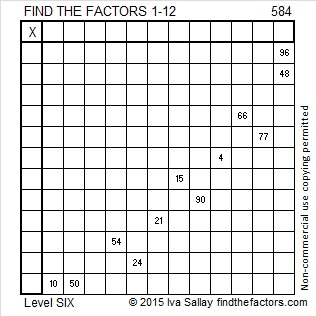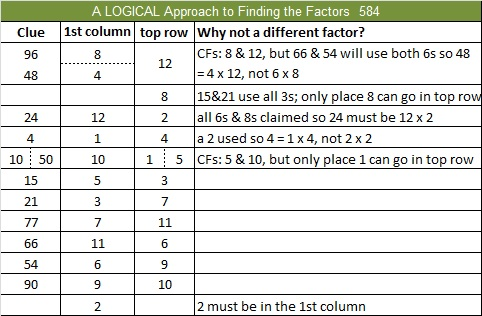# 584 and Level 6

584 is the hypotenuse of the Pythagorean triple 384-440-584. Which factor of 584 is the greatest common factor of those three numbers?Print the puzzles or type the solution on this excel file: 12 Factors 2015-08-10

—————————————————————————————————

• 584 is a composite number.
• Prime factorization: 584 = 2 x 2 x 2 x 73, which can be written 584 = (2^3) x 73
• The exponents in the prime factorization are 3 and 1. Adding one to each and multiplying we get (3 + 1)(1 + 1) = 4 x 2 = 8. Therefore 584 has exactly 8 factors.
• Factors of 584: 1, 2, 4, 8, 73, 146, 292, 584
• Factor pairs: 584 = 1 x 584, 2 x 292, 4 x 146, or 8 x 73
• Taking the factor pair with the largest square number factor, we get √584 = (√4)(√146) = 2√146 ≈ 24.16609—————————————————————————————————